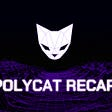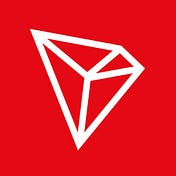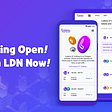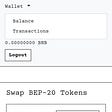# Introduction

Scaling up any blockchain’s network may lead to delays on transaction confirmation, as seen in the Ethereum and Bitcoin networks. To ensure smooth network operation, the TRON network grants every account a free pool of `Bandwidth Points` for free transactions every 24 hours. To engage in transactions more frequently requires freezing TRX for additional bandwidth points, or paying the fee in TRX. Transactions are transmitted and stored in the network in byte arrays. Bandwidth points consumed in a transaction equals the size of its byte array. If the length of a byte array is 200 then the transaction consumes 200 bandwidth points.

# Bandwidth Points Calculation

Bandwidth points are the number of usable bytes for an account per day. In any given period of time, the entire network handles a fixed amount of bandwidth. The ratio of bandwidth points in an account to the bandwidth capacity of TRON’s network equals the ratio of frozen balance in an account to frozen balance on the entire network. For example, if the frozen asset on the entire network totals 1,000,000 TRX and one given account froze 1,000 TRX (0.1% of total TRX frozen), then the account can perform roughly 300 transactions per day. Note: Since the amount of frozen asset on the entire network and for a certain account are subject to change, bandwidth points held by an account isn’t always fixed.

# Normal Transaction

In a normal transaction, bandwidth points are consumed as follows:

1.Consume the bandwidth points the transaction initiator gained through frozen assets. If the Points are not enough, go to the next step.

2. Consume the transaction initiator’s free bandwidth points. If the Points are not enough, go to the next step.

3. Consume the transaction initiator’s TRX, calculated as the number of bytes in the transaction * 10 SUN.

# New Account Transaction

If a transaction requires a new account, the bandwidth Points are consumed as follows:

1. Consume the bandwidth points the transaction initiator has frozen to gain. If the initiator does not have enough bandwidth points, go to the next step.
2. Consume the transaction initiator’s TRX, which means burning 0.1TRX.

# Token Issuance Transfer

If the transaction is a token issuance transfer, the bandwidth points are consumed as follows:

1.Verify if the total free bandwidth points of the issued token asset are sufficient. Then verify if the remaining free bandwidth points of the transfer initiator are sufficient. Finally, verify if the remaining bandwidth points the token issuers have frozen to gain are sufficient. If any of these three requirements are met, then the system deducts the bandwidth points from the token issuer. If not, go to the next step.

2. Consume the bandwidth points obtained by the transaction initiator through frozen assets. If the points are not enough, go to the next step.

3. Consume the transaction initiator’s free bandwidth points. If the Points are not enough, go to the next step.

4. Consume the transaction initiator’s TRX, calculated as the number of bytes in the transaction * 10 SUN.

# Bandwidth Points Consumption

Aside from inquiries, any other type of transaction consumes bandwidth points. The bandwidth points consumption procedure is as follows:

1.If the transaction isn’t a token transfer, skip to step 2. If the transaction is a token transfer, TRON tries to charge bandwidth points from the token issuer. If the issuer does not have sufficient bandwidth points or the charge is beyond the issuer’s maximal threshold, go to step 2.

2. Charge bandwidth points from the initiator. If bandwidth points are insufficient: (1) If the transaction creates a new account, skip to step 4. (2) If the transaction does not create a new account, go to step 3.

3. Charge free bandwidth points from the initiator. If there is insufficient free bandwidth points, go to step 4.

4. TRX will be charged from and the transaction initiator and burnt. (1) For a normal transfer, it costs about 0.002 TRX. (2) If a new account is created by the transaction, it costs about 0.1 TRX. Note: When balance unfreezes, bandwidth points will be cleared since there is no more frozen TRX.

There is another exception. If the target account did not exist in a transfer, normal, or token issuance, a new account will be created and the transfer can be done. In this case, only bandwidth points consumed by account creation will be deducted. Transfer will not take up extra bandwidth points.

# Bandwidth Points Sources

There are 5000 bandwidth points for free per account per day. When an account hasn’t frozen any balance, or when its bandwidth points have run out, complimentary bandwidth points can be used. Each transaction in the TRON network is about 200 bytes, so each account enjoys about 25 transactions for free each day.

Bandwidth points can be gained in two ways:

• Freezing TRX. The quota = the TRX frozen for gaining bandwidth points / the total TRX frozen in the network for gaining bandwidth points * 43_200_000_000, which is the equally-divided fixed bandwidth points quota for all users based on the frozen TRX.
• Fixed 5,000 free TRX quota for each account.

Use `wallet/freezebalance` to freeze an account's TRX and gain bandwidth and votes.

A transaction needs to consume 200 bandwidth. The current available bandwidth is as follows:

Case 1

• Freezebandwidth: 400 Sufficient, so all consume frozen bandwidth 400–200
• Freezebandwidth: 200 Not consumed

Remaining after consumption:

• Freezebandwidth: 200
• Freebandwidth: 200

Case 2

• Freezebandwidth: 100 is insufficent, so it is not consumed
• Freebandwidth: 200 is sufficient, so all consume free bandwidth

Remaining after consumption:

• Freezebandwidth: 100
• Freebandwidth: 0

Case 3

• Freezebandwidth: 100 Insufficient
• Freebandwidth: 100 Insufficient

Both are insufficient. The transaction reports an insufficient bandwidth error and the balance remains unchanged.

# Query Account Bandwidth Points

Use an RPC call to query an account’s available bandwidth points.

• `/wallet/getaccountnet` retrieves the bandwidth points information for an account. If a key isn't present, then the value is 0.
• Shell
`{“freeNetUsed”: 557,“freeNetLimit”: 5000,“NetUsed”: 353,“NetLimit”: 5239157853,“TotalNetLimit”: 43200000000,“TotalNetWeight”: 41228}`

# Bandwidth Points Calculator

For estimating BP from freezing TRX and maximum BP limit, please use Tron Stationbandwidth points tool to calculate.

# Automatic Recovery of Bandwidth Points

If the total network locks funds and the account locked funds remain unchanged, the account bandwidth points consumed amount is proportionally attenuated with time and is attenuated to 0 at 24 hours. For example, between time T1 and T1+12 hours, the user consumes U amount of account bandwidth points. The account then uses bandwidth u again, resulting in a consumption of U/2 + u bandwidth points. The formula follows below:

Bandwidth Points Restoration Equation

Thus, the user consumed bandwidth value is reset to 0 every 24 hours.

--

--

--

## More from TRON DAO

Love podcasts or audiobooks? Learn on the go with our new app.

## First Rubic Weekly Report 10/02## Week 7: Everything is going according to the plan (seriously)## The Zed Experience## SherpaX Crowdloan Tutorial## Introduction to Tevaera## COCOS Token Reduction Plan Annoucement## Blockchain Man and Woman will have to wait## CCTIP Lists Tokens of Polygon## Ludena Staking is Now Live!!## Sending BNB to Z3NS Wallet